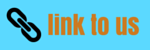# Impulse and Linear momentum

### (1) Introduction

• We have already studied about the newton's laws of motion and about their application
• It becomes difficult to use Newton's law of motion as it is while studying complex problems like collision of two objects,motion of the molecules of the gas,rocket propulsion system etc
• Thus a further study of newton's law is required to find some theorem or principles which are direct consequences of Newton's law
• We have already studied one such principle which is principle of conservation of energy.Here in this chapter we will define momentum and learn about the principle of conservation of momentum .
• Thus we begin this chapter with the concept of impluse and momentum which like work and energy are developed from Newton's law of motion

### (2) Impulse and Linear momentum

• To explain the terms impulse and momentum consider a particle of mass m is moving along x-axis under the action of constant force F as shown below in the figure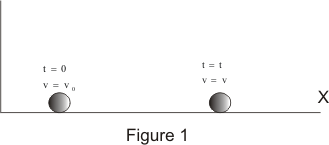• If at time t=0 ,velocity of the particle is v0 then at any time t velocity of particle is given by the equation
v = v0 + at
where a = F/m
can be determined from the newton's second law of motion .Putting value of acceleration in above equation\
we get
mv = mv0 + Ft
or
Ft = mv - mv0                   -(1)
• right side of the equation Ft, is the product of force and the time during which the force acts and is known as the impluse
Thus
Impulse= Ft
• If a constant force acts on a body during a time from t1 and t2,then impulse of the force is
I = F(t2-t1)                  -(2)
Thus impulse recieved during an impact is defined as the product of the force and time interval during which it acts
• Again consider left hand side of the equation (1) which is the difference of the product of mass and velocity of the particle at two different times t=0 and t=t
• This product of mass and velocity is known as linear momentum and is represented by the symbol p. Mathematically
p = mv                  --(3)
• physically equation (1) states that the impulse of force from time t=0 to t=t is equal to the change in linear momentum during

• If at time t1 velocity of the particle is v1 and at time t2 velocity of the particle is v2,then
F(t2-t1)=mv2-mv1                  -(4)
• so far we have considered the case of the particle moving in a straight line i.e along x-axis and quantities involved F,v, and a were all scalars
• If we call these quantities as components of the vectors F,v and a along x-axis and generalize the definations of momentum and impulse so that the motion now is not constrained along one -direction ,Thus we got
Impulse=I=F(t2-t1)                  -(5)
Linear momentum=p=mv                  -(6)
where
I=Ixi+Iyj+Izk
F=Fxi+Fyj+Fzk
p=pxi+pyj+pzk
v=vxi+vyj+vzk
are expressed in terms of their components along x,y and z axis and also in terms of unit vectors
• On generalizing equation (4) using respective vectors quantities we get the equation
F(t2-t1) =mv2-mv1                  -(7)
• So far while discussing Impulse and momentum we have considered force acting on particle is constant in direction and maagnitude
• In general ,the magnitude of the force may vary with time or both the direction and magnitude may vary with time
• Consider a particle of mass m moving in a three-dimensional space and is acted upon by the varying resultant force F. Now from newtons second law of motion we know that
F=m(dv/dt)
or Fdt=mdv
• If at time t1 velocity of the particle is v1 and at time t2 velocity of the particle is v2,then from above equation we have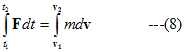• Integral on the left hand side of the equation (8) is the impulse of the force F in the time interval (t2-t1) and is a vector quantity,Thus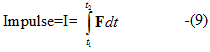Above integral can be calculated easily if the Force F is some known function of time t i.e.,
F=F(t)

• Integral on the right side is when evaluated gives the product of the mass of the particle and change in the velocity of the particle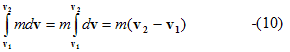• using equation (9) and (10) to rewrite the equation (8) we get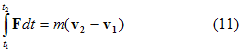• Equivalent equations of equation (11) for particle moving in space are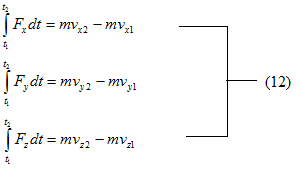• Thus we conclude that impulse of force F during the time interval t2-t1 is equal to the change in the linear momentum of the body on which its acts

• SI units of impulse is Ns or Kgms-1
Watch this tutorial for more information on How to solve momentum/Impulse problems